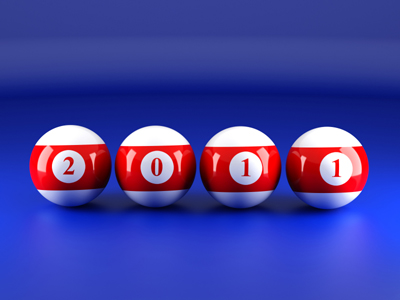In the number 2,011 the digit '2' represents two thousands.

# Place Value (Year 4)

As children progress through KS2 they will learn more about the place values of digits in Maths. As well as understanding the different values of digits depending on their position (ones, tens and hundreds), in Year Four children will be exposed to larger numbers including thousands. They will also be expected to know the equivalent values of these positions, for example that ten tens are the same as one hundred or that twenty hundreds are the same as two thousands. Finally, they should also be able to write these numbers in the form of words.

Place values are split into thousands, hundreds, tens, ones, tenths and hundredths. In our number system we know the value of a digit in a number by its position in that number - one digit represents ones, two represent tens and ones etc.

Have a go at the following quiz to see how much you know about place value. This quiz is intended for children aged 8-9.

1.
Which is the lowest number?
3 hundreds
31 tens
3 tens
3 thousands
3 tens make 30 which is the lowest number
2.
Which is the number that is equivalent to 8,025?
Eight hundred and twenty five
Eight thousand two hundred and five
Eight thousand two hundred and fifty
Eight thousand and twenty five
8,025 has 8 thousands, 0 hundreds, 2 tens and 5 ones
3.
What does the digit 2 represent in 3,204?
2 ones
2 tens
2 hundreds
2 thousands
3,204 has 3 thousands, 2 hundreds, 0 tens and 4 ones
4.
What needs to be added to change 4,328 to 4,628?
3
30
300
3,000
There is a 3 in the hundreds column that needs to change to a 6 therefore we add 3 hundreds
5.
What is the figure 1 worth in the number 4,102?
1 one
1 ten
1 hundred
1 thousand
4,102 has 4 thousands, 1 hundred, 0 tens and 2 ones
6.
What is the figure 8 worth in the number 1,482?
8 ones
8 tens
8 hundreds
8 thousands
8 tens is the same as eighty
7.
What does the digit 4 represent in 2,498?
4 ones
4 tens
4 hundreds
4 thousands
The four is in the hundred position so therefore it represents 4 hundreds
8.
Which is 2,150 in words?
Two thousand one hundred and fifty
Two thousand one hundred and five
Two hundred and fifteen
Two thousand and fifteen
2,150 has 2 thousands, 1 hundred, 5 tens and 0 ones
9.
Which is the number that is equivalent to 3,818?
Three hundred and eighty eight
Three thousand and eighteen
Three thousand eight hundred and eighteen
Three hundred thousand eight hundred and eighteen
3,818 has 3 thousands, 8 hundreds, 1 ten and 8 ones
10.
Which is 1,455 in words?
One hundred and forty five
One thousand, four hundred and fifty five
One thousand, four hundred and fifty
One thousand and fifty five
1,455 has 1 thousand, 4 hundreds, 5 tens and 5 ones